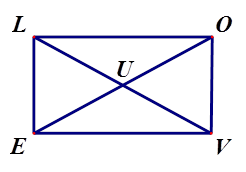# Rectangles Warm-Up

Author:
powere
Topic:
Rectangle

1. List the properties of rectangles.2. Given quadrilateral LOVE is a rectangle, find the value of x if m<LEO = 3x+4 and m<VEO = 2x-1.

Select all that apply
• A
• B
• C
• D3. Given quadrilateral LOVE is a rectangle, fine the value of x if OE = 18x-5 and LV = 13x+14.

Select all that apply
• A
• B
• C
• D